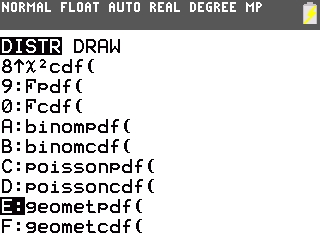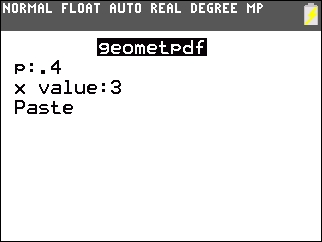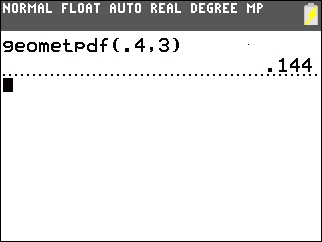# Knowledge Base

## Solution 35011: Calculating a Geometric Distribution on the TI-84 Plus Family of Graphing Calculators.

### How do I calculate a Geometric Distribution on the TI-84 Plus family of graphing calculators?

The example below will demonstrate how to use the Geometric Distribution command.

Assume that 40 percent of a large lot of electrical components are from the Donut-Tech company. With components being selected at random, what is the probability that the components from Donut-Tech will be selected on a 3rd pic?

• Press [2nd][DIST]
• Press [Alpha] [SIN] to select geometpdf( by using Alpha E.• Press [.]  [Down]  [Down]• Press [ENTER] twice.